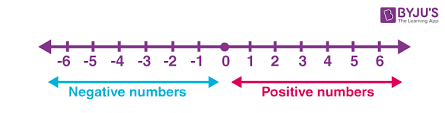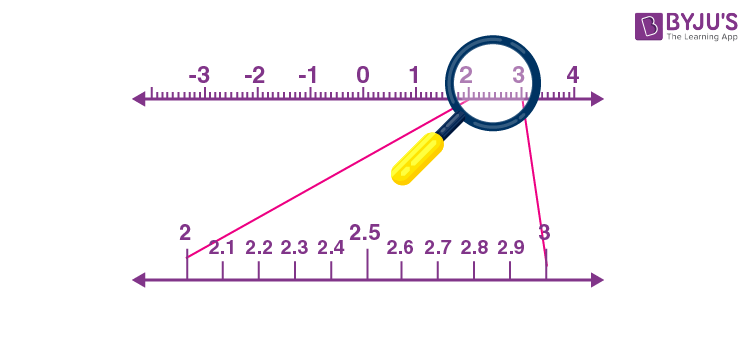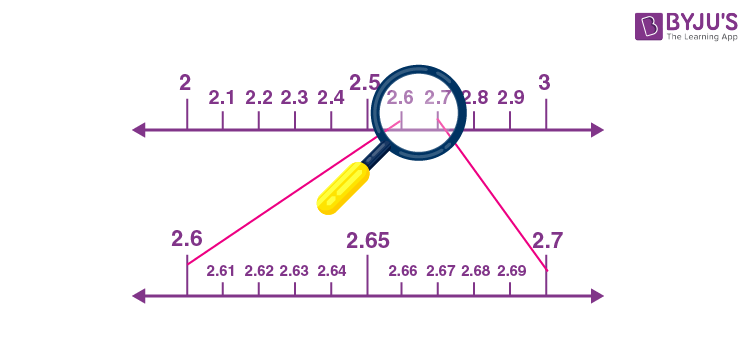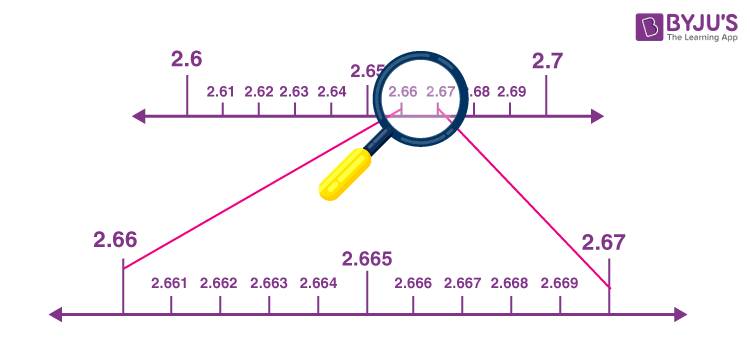# Representing Real Numbers on the Number Line

In Mathematics, a number line is a straight line, which represents the integers in equal intervals. The number line extends infinitely in both directions, and it contains both positive and negative integers. In this article, we are going to learn how to represent the real numbers on the number line with a complete explanation.

Table of Content:

## Real Number Line

The real number is defined as the union of a set of rational numbers and the set of irrational numbers. The real numbers include natural numbers, whole numbers, integer, rational and irrational numbers. We can represent all these types of numbers in the number line. The complex number is not represented using the number line as it is not included in the real number system.The Real number line is just a number line. The point associated with the real number line is called the coordinate. The number “0” represents the origin. All the positive numbers are represented on the right side of the origin, whereas all the negative numbers are represented on the left side of the origin.

## How to Represent the Real Numbers on the Number Line

Go through the following steps to represent the real numbers on the number line.

Step 1: Draw a horizontal line with arrows on both sides. Mark the origin “0” anywhere on the horizontal line.

Step 2: Mark the real numbers on the number line of equal lengths on both sides with a definite scale.

Step 3: Mark the positive numbers on the right side of the origin and negative numbers on the left side of the origin.

Step 4: If the given point is large, adjust the scale accordingly.

Step 5: If the given number is a rational or irrational number, convert them into a decimal format and mark the points using the process of successive magnification.

Note: The process of visualization of numbers on the number line using the magnifying glass is called the process of successive magnification.

Assume that, we want to locate the point 2.665 on the number line.

We know that point 2.665 lies on the number line between 2 and 3.In between 2 and 3, there are 10 equal parts, say 2.1, 2,2, 2.3, and so on. In order to locate the 2.665 exactly, again focus on the points between 2.6 and 2.7, as 2.665 is located in between.Since 2.665 is located between 2.66 and 2.67, again focus on these points.Thus, point 2.665 is located on the number line using the process of successive magnification. So, with the help of this method, one can locate the point by a sufficient successive magnification process to visualize the representation of real numbers (rational and irrational numbers) on the number line.

Also, read: Rational and Irrational Numbers.

### Practice Problems

Plot the following real numbers on the number line:

1. 2.5
2. 2.67
3. 5.37
4. 3.765
5. 4.55

To learn more Maths-related and class-wise concepts, visit BYJU’S – The Learning App and learn the concepts easily by exploring more videos.

## Frequently Asked Questions on Representing Real Number on the Number Line

### What is meant by a real number line?

The real number line is a number line whose points are the real numbers. The real number line is a horizontal line with arrows on both sides. It consists of the origin “0”. All the positive numbers are represented on the right side of the origin and all the negative numbers are represented on the left side of the origin, with a definite scale.

### Mention the types of numbers which can be represented on the number line?

Real numbers can be represented using the number line. All the natural numbers, whole numbers, integers, rational numbers and irrational numbers can be represented on the number line.

### Can we represent complex numbers on the number line?

No, it is not possible to represent the complex numbers on the number line.

### Which process is used to locate the rational and irrational numbers on the number line?

To locate the rational and irrational numbers on the number line, the process of successive magnification is used.

### Define the process of successive magnification.

The process of successive magnification is the visualization of the representation of numbers on the number line through the magnifying glass.